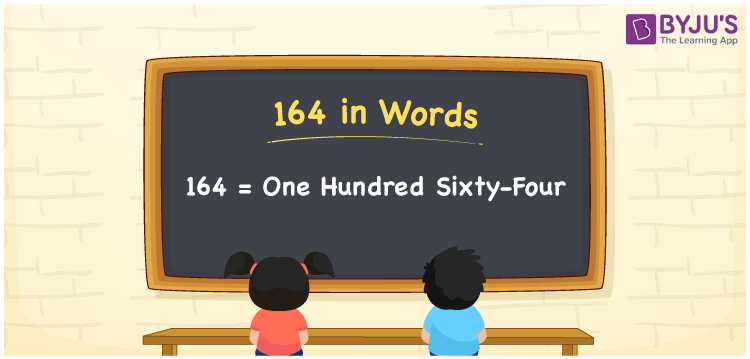# 164 in Words

164 in words is written as One hundred sixty-four. In both the International System of Numerals and the Indian System of Numerals, 164 is written as One hundred sixty-four. The number 164 is a Cardinal Number as it represents some quantity. For example, “there are 164 chairs in this room”.

 164 in Words One hundred sixty-four One hundred sixty-four in Number 164

## 164 in English Words

We write 164 in English Words using the letters of the English alphabet. Therefore, we read 164 in English as “One hundred sixty-four.”## How to Write 164 in Words?

To write 164 in words, we shall use the place value chart. In the place value chart, write 1 in the hundreds, 6 in the tens, and 4 in the ones, respectively. Now let us make a place value chart to write the number 164 in words.

 Hundreds Tens Ones 1 6 4

Thus, we can write the expanded form as

1 × Hundred + 6 × Ten + 4 × One

= 1 × 100 + 6 × 10 + 4 × 1

= 100 + 60 + 4

= 164

= One hundred sixty-four.

164 is a natural number, the successor of 163 and the predecessor of 165.

164 in words – One hundred sixty-four

• Is 164 an odd number? – No
• Is 164 an even number? – Yes
• Is 164 a perfect square number? – No
• Is 164 a perfect cube number? – No
• Is 164 a prime number? – No
• Is 164 a composite number? – Yes

## Frequently Asked Questions on 164 in Words

Q1

### How to write 164 in words?

164 in words is written as One hundred sixty-four.
Q2

### How to write 164 in the International and Indian System of Numerals?

In both, the system of numerals, 164 in words, is written as One hundred sixty-four.
Q3

### What are the three consecutive natural numbers after 164?

The three consecutive natural numbers after 164 are 165, 166 and 167.